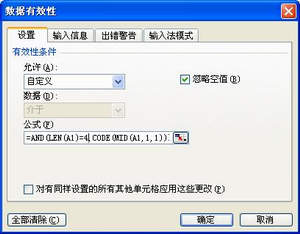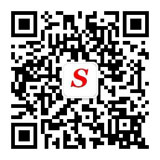﻿ Excel使用有效性分辨中英文-Storm_Center

# Excel使用有效性分辨中英文

又是同事要求，说是要在Excel文档中对输入的内容进行检查，要求符合“两个中文+两个数字”的格式。这个检查可以用有效性（菜单－数据－有效性）来做，具体检查可以使用code函数，得到目标字串中第一个字符的ANSI码，汉字是双字节的，ANSI码范围高字节从B0-F7，低字节从A1-FE，所以远大于数字与英文的这ANSI码值，可以用此来分辨中文、英文和数字。做法如下，在“数据有效性”的设置－有效性条件－允许中选择“自定义”，在公式上填写如下内容：

=AND(LEN(A1)=4,CODE(MID(A1,1,1))>45216,CODE(MID(A1,2,1))>45216,CODE(MID(A1,3,1))>47,CODE(MID(A1,3,1))<58,CODE(MID(A1,4,1))>47,CODE(MID(A1,4,1))<58)后来想想，反正只是为了给个提醒，没必要这么精确，一个一个字符检查多麻烦（幸好本例中检查的字符不多），可以改用模糊一点的方法，公式改用LENB和LEN函数，LENB得出的是字符串的字节数，而LEN返回的字符数，汉字是两个字节，英文与数字是单字节的，这样只要检查总字符数加前两个字符的字节数就可以了，公式改成

=AND(LEN(A1)=4,LENB(LEFT(A1,2))=4,LENB(RIGHT(A1,2))=2)　或者　=AND(LEN(A1)=4,LENB(A1)=6,LENB(LEFT(A1,2))=4)

至于这个有效性，还有其它功能，比如，在“允许”那选择“序列”，再在后面的“来源”上输入具体值（用逗号分割），就可以做成下列菜单的形式，很好玩的。如果要用公式做判断，要写成逻辑判断式，开始同事为了给我演示有效性的用法，想做个要求单元格中的输入值为1的限制，在公式直接写=1或1，就是不成功，其实应该是写成A1=1的形式，其实就是一个逻辑判断式，就象前面两组公式一样：LEN(A1)=4（AND本来就是逻辑函数）。

>> 除非说明均为原创，如转载请注明来源于http://www.stormcn.cn/post/1210.html

◎欢迎参与讨论，请在这里发表您的看法、交流您的观点。

•微信订阅